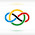### 2013 IMO #4

Let $ABC$ be an acute triangle with orthocenter $H$, and let $W$ be a point on the side $BC$, lying strictly between $B$ and $C$. The points $M$ and $N$ are the feet of the altitudes from $B$ and $C$, respectively. Denote by $\omega_1$ is the circumcircle of $BWN$, and let $X$ be the point on $\omega_1$ such that $WX$ is a diameter of $\omega_1$. Analogously, denote by $\omega_2$ the circumcircle of triangle $CWM$, and let $Y$ be the point such that $WY$ is a diameter of $\omega_2$. Prove that $X,Y$ and $H$ are collinear.

Solution 1:
Let $\omega_1$ and $\omega_2$ meet again at $P$. Then by the Miquel Point, $P$ is on the circumcircle of $\triangle{AMN}$, which has diameter $H$. Thus, $\measuredangle{APH}=\frac{\pi}{2}$. In particular, $H$ lies on the line through $P$ perpendicular to $AP$. But note that$\measuredangle{XPW}=\frac{\pi}{2}=\measuredangle{YPW}$because $WX$ and $WY$ are diameters of $\omega_1$ and $\omega_2$, respectively, so $X$ and $Y$ lie on the line through $P$ perpendicular to $WP$.

We now claim that $A$, $P$, and $W$ are collinear. It suffices to prove that $A$ is on the radical axis of $\omega_1$ and $\omega_2$. But this is true because $A$ is the radical center of $\omega_1$, $\omega_2$, and the circumcircle of cyclic quadrilateral $BNMC$ (because it is $BN\cap CM$). Thus, $A$, $P$, and $W$ are collinear.

Now, $H$, $X$, and $Y$ all lie on the line through $P$ perpendicular to $WP$, so $X$, $Y$, and $H$ are collinear, as desired. $\square$

Solution 2:
Let $P$ be the second intersection of $\omega_1, \omega_2$. Then $P$ is the Miquel point of $\triangle MNW$ w.r.t $\triangle ABC$, so $AMPHN$ is cyclic. Now $BX \parallel AH$, so $X, H, P$ are collinear by Reim's theorem. Similarly, $H,P,Y$ are collinear, and the result follows. $\square$

## Notice:

I will rarely be posting from now till July 4th, because I will be on vacation. However, if you have any questions regarding my previous solutions then you can always contact me (see contact info on sidebar) and I will most likely be able to respond in a day or two. Have a nice summer!

1.Anonymous6/09/2022

It's amazing to see how far this guy has come. See you after the summer!

2.Anonymous6/10/2022

Have a gret vacation! just curiuos, where are you going?

1.India!